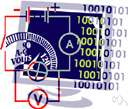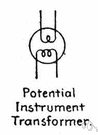voltage

(redirected from Difference of electric potential)
Also found in: Thesaurus, Medical, Encyclopedia.
Related to Difference of electric potential: electric field, electric current, Electric potential energy

volt·age

(vōl′tĭj)
n.
A measure of the difference in electric potential between two points in space, a material, or an electric circuit, expressed in volts.

voltage

(ˈvəʊltɪdʒ)
n
(General Physics) an electromotive force or potential difference expressed in volts

volt•age

(ˈvoʊl tɪdʒ)

n.
electromotive force or potential difference expressed in volts.
[1885–90]

volt·age

(vōl′tĭj)
Electromotive force expressed in volts. A current of high voltage is used to transmit electric power over long distances.
ThesaurusAntonymsRelated WordsSynonymsLegend:
 Noun 1voltage - the rate at which energy is drawn from a source that produces a flow of electricity in a circuit; expressed in voltselectrical phenomenon - a physical phenomenon involving electricity 2voltage - the difference in electrical charge between two points in a circuit expressed in voltselectrical phenomenon - a physical phenomenon involving electricityevoked potential - the electrical response of the central nervous system produced by an external stimulus; "he measured evoked potentials with an electroencephalogram"resting potential - the potential difference between the two sides of the membrane of a nerve cell when the cell is not conducting an impulse
Translations
جُهْدفُلْطِيَّه: جُهْد كَهْرُبائي
napětí
spændingvoltstyrke
pinge
jännite
voltaža
rafspenna

전압
spänning
แรงดันไฟฟ้าที่มีหน่วยเป็นโวลต์
điện áp

[ˈvəʊltɪdʒ] N

[ˈvəʊltɪdʒ] n
low voltage →
high voltage →

voltage

nSpannung f; what voltage is this cable?wie viel Volt hat dieses Kabel?

voltage

[ˈvəʊltɪdʒ] n
high/low voltage →

volt

(vəult) noun
(often abbreviated to V) the unit used in measuring the force driving electricity through a circuit, or the strength of an electric current.
ˈvoltage (-tidʒ) noun
(a) force measured in volts. Low voltage reduces the current, making the lights burn dimly.

voltage

napětí spænding jännite voltaža 電圧 전압 spänning แรงดันไฟฟ้าที่มีหน่วยเป็นโวลต์ điện áp
References in periodicals archive ?
x,m] is the difference of electric potential between two vertices of one of the four edges in the x-direction and [l.
When a certain current intensity i (in mA) flows through the subsoil another couple of electrodes (M and N) register the difference of electric potential V(in mV) generated in the subsoil.
The operation of measuring values of the difference of electric potential throughout a profile is repeated in other parallel profiles and separated to each other in a fixed distance covering in this way, the surface of interest and keeping constant the electrical current i(5mAinthiscase).

Site: Follow: Share:
Open / Close